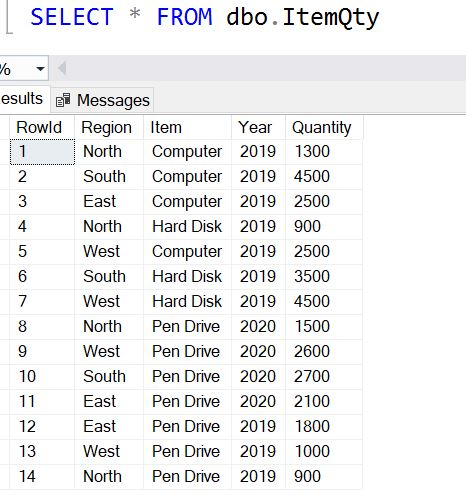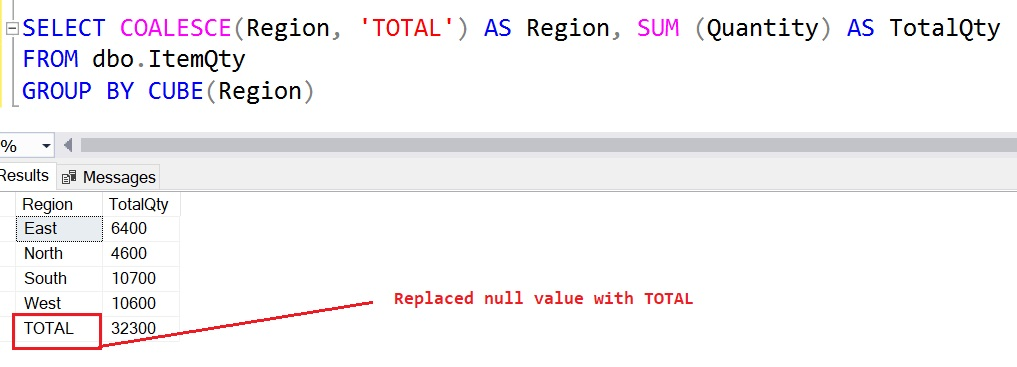Home » SQL Server CUBE

# SQL Server CUBECUBE operator is used to calculate subtotals and grand total for all combinations of grouping columns specified in the GROUP BY  clause.

It is similar to the operators which extend the functionality of GROUP BY clauses by calculating subtotals and grand totals for a set of columns.

CUBE uses the same functionality of ROLLUP Operator but it returns the subtotal and grand total for all combinations of grouping columns specified in the GROUP BY clause.

#### SYNTAX

```SELECT
col1, col2, AggregateFunction(col3)
FROM
table_name
GROUP BY CUBE(col1, col2);```

Lets look at an example of CUBE in SQL Server.

To demonstrate this first we create a sample table named ItemQty which contains the details of purchased Item and insert some records in table.

```CREATE TABLE dbo.ItemQty
(
RowId INT IDENTITY(1,1),
Region VARCHAR(50),
Item VARCHAR(50),
Year INT,
Quantity INT
)
INSERT INTO dbo.ItemQty(Region, Item, Year, Quantity)
VALUES('North', 'Computer', 2019, 1300),
('South', 'Computer', 2019, 4500),
('East', 'Computer', 2019, 2500),
('North', 'Hard Disk', 2019, 900),
('West', 'Computer', 2019, 2500),
('South', 'Hard Disk', 2019, 3500),
('West', 'Hard Disk', 2019, 4500),
('North', 'Pen Drive', 2020, 1500),
('West', 'Pen Drive', 2020, 2600),
('South', 'Pen Drive', 2020, 2700),
('East', 'Pen Drive', 2020, 2100),
('East', 'Pen Drive', 2019, 1800),
('West', 'Pen Drive', 2019, 1000),
('North', 'Pen Drive', 2019, 900)```

As you can see now we have a table named ItemQty as shown below.

`SELECT * FROM dbo.ItemQty`Lets, see how CUBE operator returns the subtotal and grand total for all combinations of grouping columns specified in the GROUP BY clause.

Suppose, you want to display the total purchased quantity by region, then you can use the following query .

```SELECT Region, SUM (Quantity) AS TotalQty
FROM dbo.ItemQty
GROUP BY Region```As you can see, it returns total Quantities by Region.

To display the Over all total quantity by Region in above result,  then you can use the CUBE operator in the GROUP BY clause as given below.

```SELECT Region, SUM (Quantity) AS TotalQty
FROM dbo.ItemQty
GROUP BY CUBE(Region)

```As you can see, CUBE operator adds a Over all total quantity row with a null value in the Region column.  You can also replace null value with meaningful name.

Lets replace the null value with ‘Total’ to make an output more meaningful.

```SELECT COALESCE(Region, 'TOTAL') AS Region, SUM (Quantity) AS TotalQty
FROM dbo.ItemQty
GROUP BY CUBE(Region)```In order to display total quantity by Region and Item, use the following query.

```SELECT Region, Item, SUM (Quantity) AS TotalQty
FROM dbo.ItemQty
GROUP BY Region, Item```But in case, if you want display subtotals and Grand Total of quantities by Region and Item, then you can use CUBE in above query as shown below.

```SELECT Region, Item, SUM (Quantity) AS TotalQty
FROM dbo.ItemQty
GROUP BY CUBE(Region, Item)
ORDER BY Region, Item```You can see, Subtotal and Grand totals in result set.

Lets understand the output of query:

1st row displays Grand Total.

2nd, 3rd and 4th rows displays Item wise Quantity subtotals.

5th, 8th, 12th and 16th rows displays the Region wise Quantity subtotals.

6th, 7th, 8th, 9th, 10th,11th, 13th, 14th, 15th, 17th, 18th, and 19th rows displays the Region and Item wise subtotals of quantity.

As you know that CUBES returns the Subtotal and Grand total for all the combination of grouping columns specified in the GROUP BY clause, so it is required that all the select columns must be passed in group by cube clause other wise it will return an error.

Lets produce a case when you get an error.

As you can see in below query, there are two columns in select statement that is Region and Item but Item column is not passed in Group By clause, so it gives an error .

```SELECT Region, Item, SUM (Quantity) AS TotalQty
FROM dbo.ItemQty
GROUP BY CUBE(Region)
ORDER BY Region, Item```